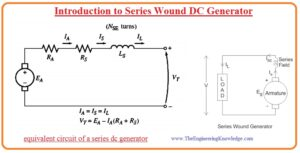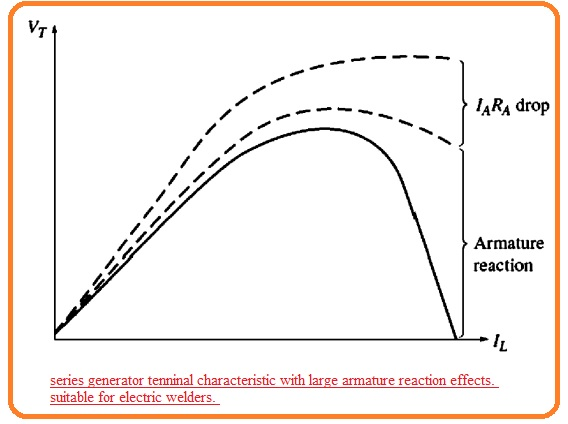Hi, reader welcome to the new post. In this post, we will have a detailed look at the Characteristics of Series Wound DC Generator. The generator is an electrical device used to convert mechanical power into electrical power. such generator that generates ac power called ac generator. It has a further two subtypes synchronous generator and induction generator. The generator that converts mechanical power in the dc power called a dc generator.

The basic principle of any generator is based on the Faraday law of electromagnetic induction. In this post, we will discuss the series dc generator and its related parameters. so let’s get started with Characteristics of Series Wound DC Generator.

## Introduction to Series Wound DC Generator

• The series dc generator has such a configuration of field and armature windings that both are linked in series combination.
• The current in armature windings is larger than the shunt field windings while in series combination less number of turns are used in wire and dia of wire used in series is larger than the wire used in shunt windings.
• Since mmf has this equation F=NI the similar value MMF can be generated through the less number of turns than high current produced from numerous turns having less current.
• As the full load current passes through it the resistance in the series windings is less. The equivalent circuit of the series dc generator can be seen here.• In this circuitry, the armature current field current and line current have similar values. After applying KVL to this circuit we have.

VT=EA-IA(RA+RS)

#### Terminal Characteristic of a Series Generator

• The magnetization curve of series dc generator is like the magnetization curve of other types of generators.
• As no load is linked to the generator so field current also not exist therefore Vt decreases to such less value given by the residual flux in the generator.
• With the increment in load file current also increases, therefore internally generated voltage increases.
• The IA(RA+RS) also increases but at the start increment in EA becomes very fast then the IA(iRA+Rs) losses increment therefore VT rises.
• After that generator becomes saturated and the internal generated voltage becomes constant. In this condition, the resistance losses are the dominant factor and VT goes to decrement.
• The category of characteristics can seen here.• It is certain that this generator will make a constant voltage source. The voltage regulation is a negative number.
• The series generator used in certain applications for such cases where the steep voltage curve of the machine can be shown.
• On application is arc welding. Series generators used in arc welding are created to get a high armature reaction that provides terminal characterize such as shown here.• Note that when welding electrodes do connect to one another before start welding the huge current will move.
• As the operating person separates the welding electrodes there is high steep rises in the voltage of the generator and the value of the current is constant.
• The voltage makes sure that a welding arc is sustaining through the air among the electrodes.

That is a detailed post about the series dc generator. i tried my level best to explain each and every parameter related to this generator. If you have any further query ask in the comments. thanks for reading. Have a nice day.